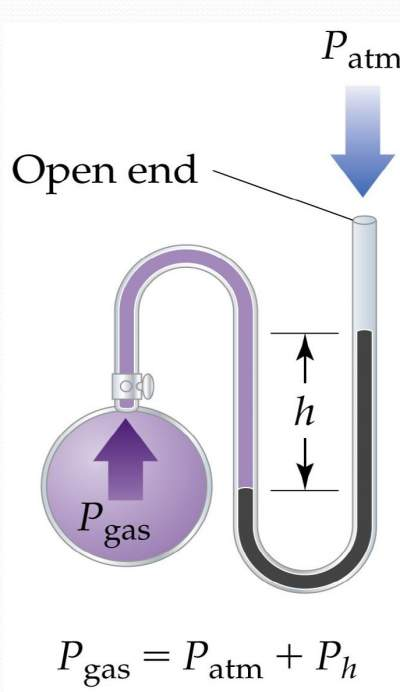# Characteristics of gases

## Introduction

• Gases are highly compressible and occupy the full volume of their containers.
• When a gas is subjected to:
• High pressure, its volume decreases.
• High temperature, its volume increases.
• Gases always form homogeneous mixtureswith other gases.
Example: Air

### Pressure

It is the force acting on an object per unit area. P = F / A
Example:
Gravity exerts a force on the earth's atmosphere.
If a column of air 1 m2 in cross section exerts a force of 105 N.
Therefore, the pressure of a 1 m2 Column of air is 100 kPa (kilo Pascals)
SI Units of pressure: 1 N = 1 kg•m/s2; 1 Pa = 1 N/m2

• Atmospheric pressure is measured with a barometer.
• If a tube is inserted into a container of mercury (Hg) open to the atmosphere,
• Hg will rise 760 mm up the tube.
• ONE Atmosphere is the pressure required to support 760 mm of Hg in a column.
• Units of Pressure
1 atm = 760 mm Hg = 760 torr = 1.01325 × 10 Pa = 101.325 KPa
 The pressures of gases not open to the atmosphere are measured in manometers A manometer consists of a bulb of gas attached to a U-tube containing Hg